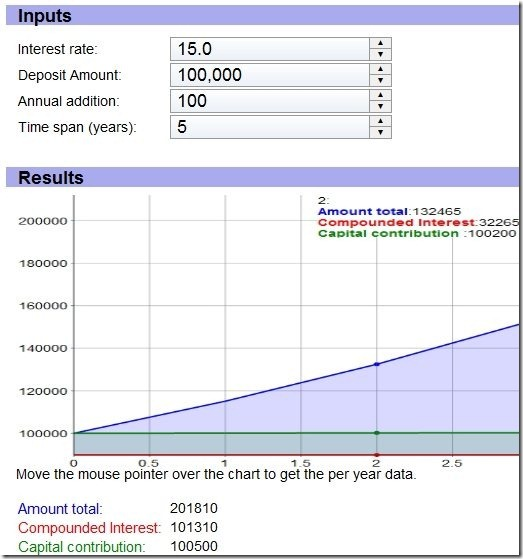# Online Compound Interest Calculator

Online compound interest calculator is a free online calculator to calculate compound interest. This is a simple to use online compound interest calculator. Just provide the starting amount, interest rate, annual addition, and span in years. It will then calculate the interest that you accumulate over the span of your deposit, and total amount that you will get at the end. This calculator also shows a nice graph that shows how your total amount changes over the span of deposit.